``````# Packages Required
library(stats)       # K-means
library(ggplot2)     # Plots
library(data.table)  # Data Tables
library(NbClust)     # Determine the Best K``````

# Introduction

K-means and hierarchical cluster analysis (HCA) are both unsupervised learning techniques that describe data with clusters.

### K-Means

After the initial step of placing K points into a data space, K-means works by iterating between two steps until a local minima is found:

• Assigning each element to one of the K centroids by closest distance
• Re-calculating the location of the centroids so that the distance between each element and the centroid is optimized (the mean)

The K-means algorithm iterates this pattern until a local minima is achieved (hill climbing). Because this algorithm does not always find the global minima, ensembling random restarts is usually employed. The important parameter in K-means is K, or number of clusters to find. Choosing the right K can require domain knowledge.

### Hierarchical Cluster Analysis

A data set with N examples can have anywhere between 1-N clusters. Hierarchical clustering starts with either 1 or N clusters, then uses a divisive (starting with 1) or agglomerative (starting with N) approach to produce N-K clusters. For an agglomerative example:

• The data starts with N clusters
• The two closest clusters combine
• This is repeated until there are K clusters (K <= N)

There are different methods to determine the distances between each cluster, these will affect the clusters produced by the algorithm:

• Single Linkage: The smallest distance between any two points between clusters
• Complete link: The largest distance between any two points between clusters
• Average linkage: The distance between the average points of all elements between clusters
• Centroid linkage: The distance between the mean point (centroid) between clusters

This approach will produce a tree type structure called a dendrogram. Each layer represents K clusters and the distance between the parents and the children give a visual representation of similarity.

# The Wholesale Customers Data Set

The data set by Abreu (2011), retrieved from the UCI machine learning repository (Lichman, 2013), contains 440 examples with 8 features representing the amount spent (monetary units) on different product categories from 3 different regions (Lisbon, Oporto, Other) and 2 channels (retail or horeca).

# Exploratory Data Analysis

The purpose of exploring the data first is to get familiar with it and to see if anything is of interest. One could argue that cluster analysis is an advanced form of EDA.

``````customer <- read.csv("wholesale-customer-data.csv") # Read in the data
str(customer)``````
``````## 'data.frame':    440 obs. of  8 variables:
##  \$ Channel         : int  2 2 2 1 2 2 2 2 1 2 ...
##  \$ Region          : int  3 3 3 3 3 3 3 3 3 3 ...
##  \$ Fresh           : int  12669 7057 6353 13265 22615 9413 12126 7579 5963 6006 ...
##  \$ Milk            : int  9656 9810 8808 1196 5410 8259 3199 4956 3648 11093 ...
##  \$ Grocery         : int  7561 9568 7684 4221 7198 5126 6975 9426 6192 18881 ...
##  \$ Frozen          : int  214 1762 2405 6404 3915 666 480 1669 425 1159 ...
##  \$ Detergents_Paper: int  2674 3293 3516 507 1777 1795 3140 3321 1716 7425 ...
##  \$ Delicassen      : int  1338 1776 7844 1788 5185 1451 545 2566 750 2098 ...``````

``lapply(customer, summary)``
``````## \$Channel
##    Min. 1st Qu.  Median    Mean 3rd Qu.    Max.
##   1.000   1.000   1.000   1.323   2.000   2.000
##
## \$Region
##    Min. 1st Qu.  Median    Mean 3rd Qu.    Max.
##   1.000   2.000   3.000   2.543   3.000   3.000
##
## \$Fresh
##    Min. 1st Qu.  Median    Mean 3rd Qu.    Max.
##       3    3128    8504   12000   16934  112151
##
## \$Milk
##    Min. 1st Qu.  Median    Mean 3rd Qu.    Max.
##      55    1533    3627    5796    7190   73498
##
## \$Grocery
##    Min. 1st Qu.  Median    Mean 3rd Qu.    Max.
##       3    2153    4756    7951   10656   92780
##
## \$Frozen
##    Min. 1st Qu.  Median    Mean 3rd Qu.    Max.
##    25.0   742.2  1526.0  3071.9  3554.2 60869.0
##
## \$Detergents_Paper
##    Min. 1st Qu.  Median    Mean 3rd Qu.    Max.
##     3.0   256.8   816.5  2881.5  3922.0 40827.0
##
## \$Delicassen
##    Min. 1st Qu.  Median    Mean 3rd Qu.    Max.
##     3.0   408.2   965.5  1524.9  1820.2 47943.0``````

The data in this example contains only 3 different units. The categorical representations of ‘Channel’ and ‘Region,’ and the monetary units (m.u.) of the rest. Exploring the Fresh category.

``````ggplot(aes(x = Fresh), data = customer) +
geom_histogram(binwidth = 1000) +
xlab('Annual Spending (m.u.)') +
ylab('Count')``````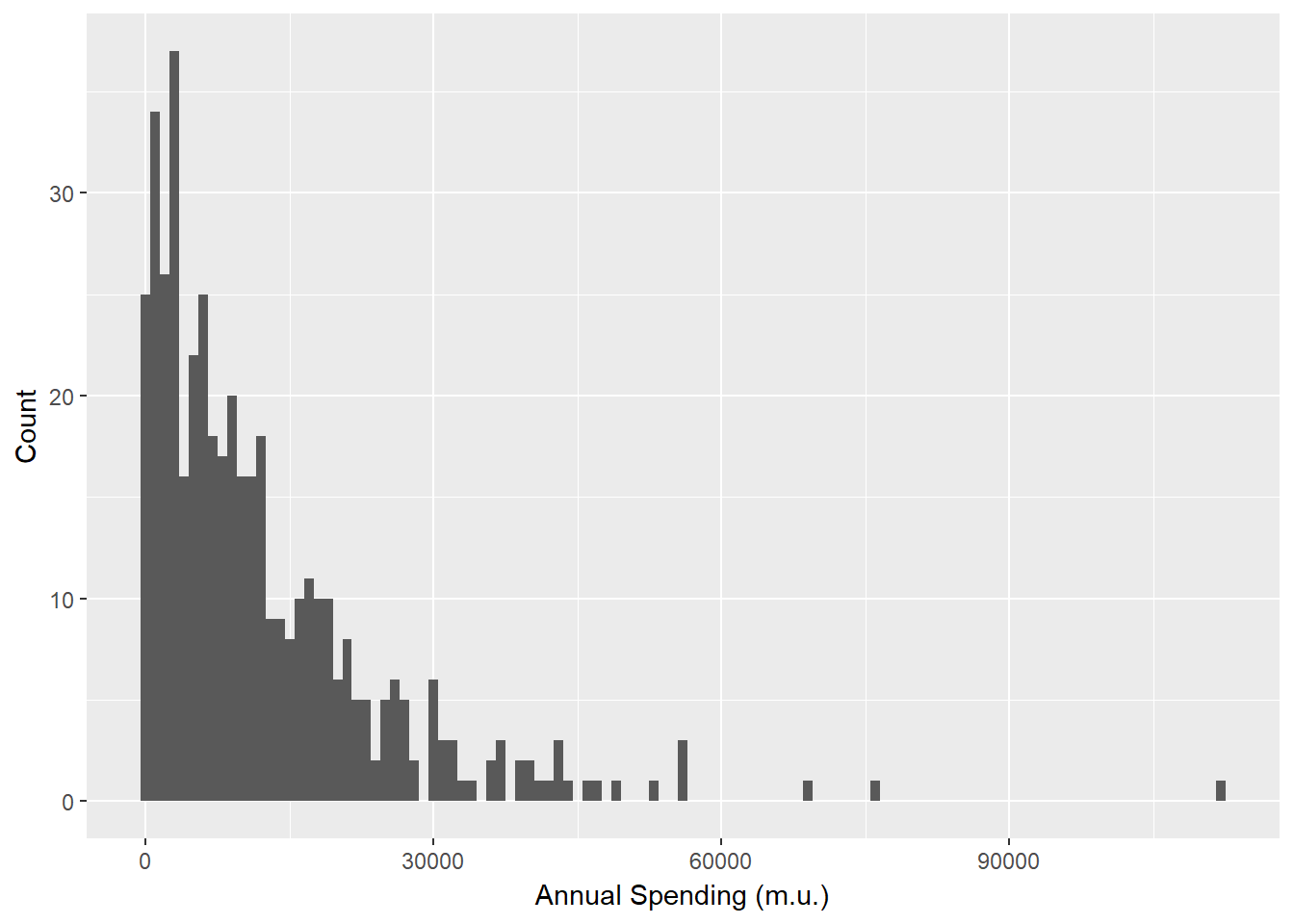Figure 1: Histogram of Spending on Fresh Products

It looks like the data are following a long tail distribution with some major outliers. Comparing this distributions with region:

``````ggplot(data = customer, aes(x = Fresh, y=..count../sum(..count..))) +
geom_freqpoly(data = customer[customer\$Region == 1, ], aes(color = "1"), binwidth = 1000) +
geom_freqpoly(data = customer[customer\$Region == 2, ], aes(color = "2"), binwidth = 1000) +
geom_freqpoly(data = customer[customer\$Region == 3, ], aes(color = "3"), binwidth = 1000) +
scale_color_discrete(name = "Region") +
xlab('Annual Spending (m.u.)') +
ylab('Proportion')``````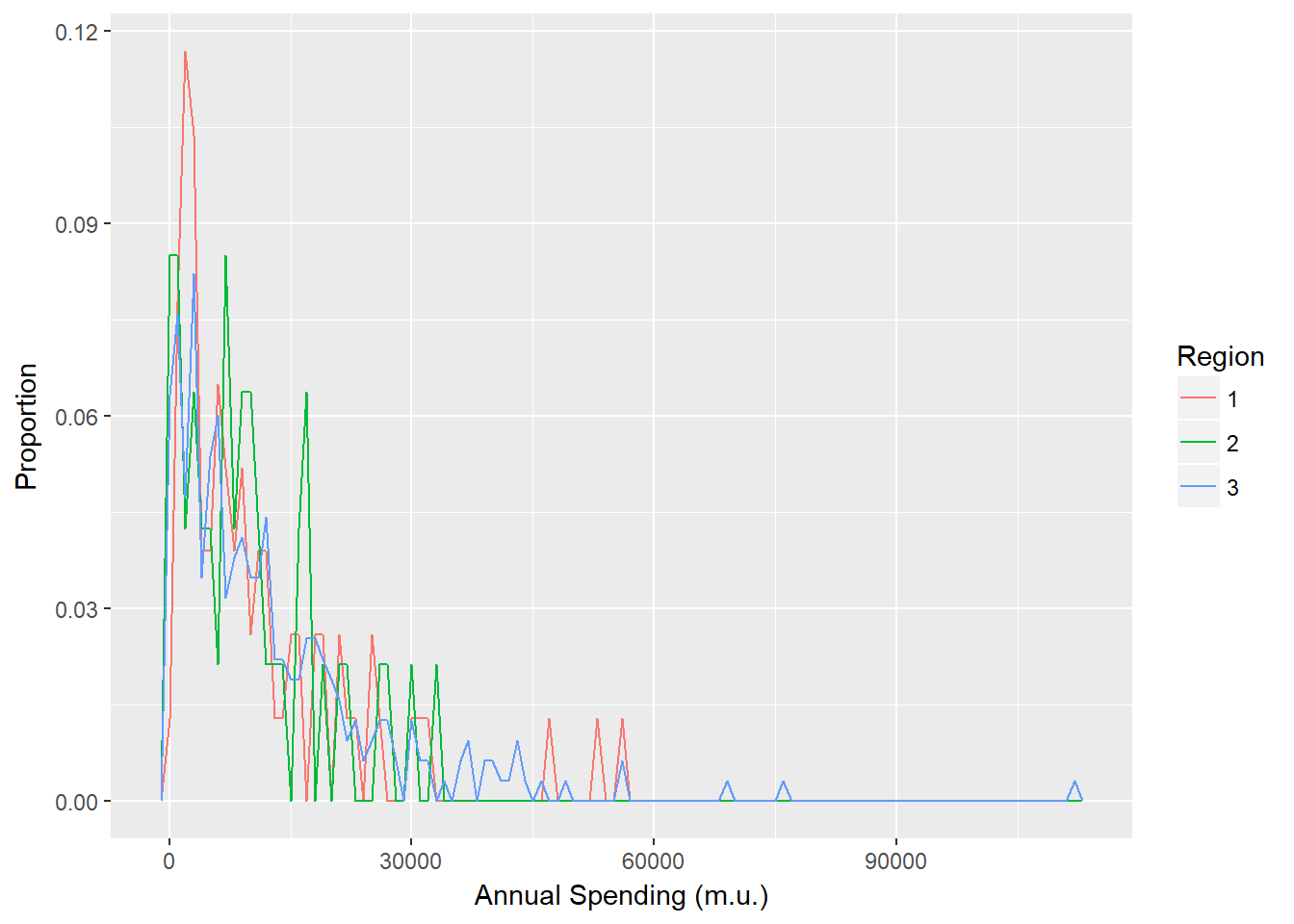Figure 2: Comparison of Spending on Fresh Products for each Region

And Channel:

``````ggplot(data = customer, aes(x = Fresh, y=..count../sum(..count..))) +
geom_freqpoly(data = customer[customer\$Channel == 1, ], aes(color = "1"), binwidth = 1000) +
geom_freqpoly(data = customer[customer\$Channel == 2, ], aes(color = "2"), binwidth = 1000) +
scale_color_discrete(name = "Channel") +
xlab('Annual Spending (m.u.)') +
ylab('Proportion')``````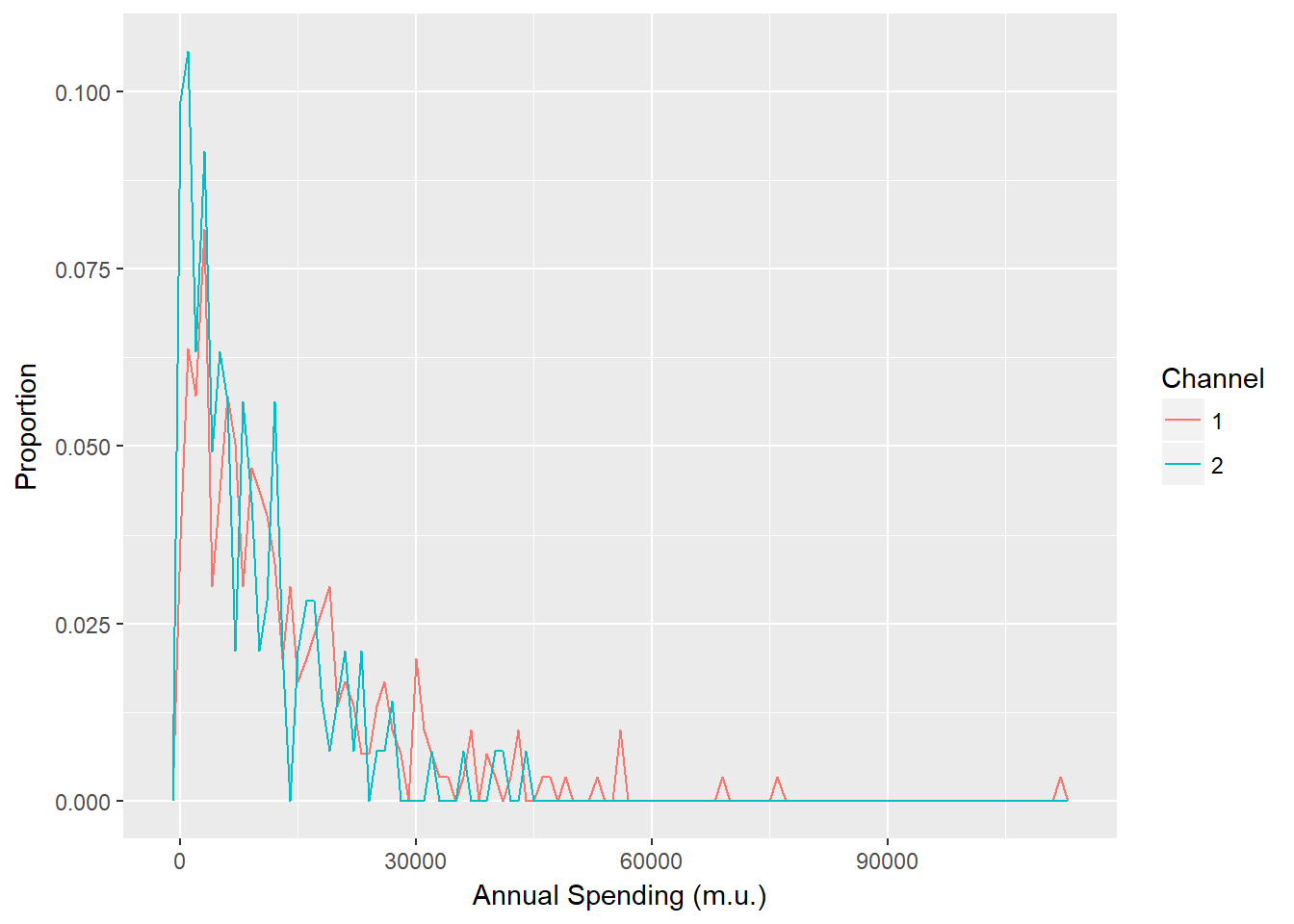Figure 3: Comparison of Spending on Fresh Products for each Channel

Perhaps some insight might be gained from comparing the total spending between regions and channels:

``````# Convert the frame to a table
customer <- data.table(customer)

# Construct a data frame of totals using the data table
fresh_prop <- customer[, sum(Fresh), by=.(Channel, Region)]
fresh_prop\$Category <- "Fresh"

milk_prop <- customer[, sum(Milk), by=.(Channel, Region)]
milk_prop\$Category <- "Milk"

grocery_prop <- customer[, sum(Grocery), by=.(Channel, Region)]
grocery_prop\$Category <- "Grocery"

frozen_prop <- customer[, sum(Frozen), by=.(Channel, Region)]
frozen_prop\$Category <- "Frozen"

detergents_paper_prop <- customer[, sum(Detergents_Paper), by=.(Channel, Region)]
detergents_paper_prop\$Category <- "Detergents_Paper"

delicassen_prop <- customer[, sum(Delicassen), by=.(Channel, Region)]
delicassen_prop\$Category <- "Delicassen"

# Combine the data tables
customer_prop <- rbind(fresh_prop, milk_prop, grocery_prop, frozen_prop, detergents_paper_prop,  delicassen_prop)

# Change 'Channel' and 'Region' to factors
customer_prop\$Channel <- as.factor(customer_prop\$Channel)
customer_prop\$Region <- as.factor(customer_prop\$Region)

# Split the data table
customer_prop_region <- subset(customer_prop, select = -Channel)
customer_prop_channel <- subset(customer_prop, select = -Region)

# Get the Channel and Region totals
channel_totals <- customer[, sum(Fresh + Milk + Grocery + Frozen + Detergents_Paper + Delicassen), by=.(Channel)]
region_totals <- customer[, sum(Fresh + Milk + Grocery + Frozen + Detergents_Paper + Delicassen), by=.(Region)]

# Calculate the amount spent per Channel/Region relative to the total
customer_prop_channel\$Prop <- 0
customer_prop_channel[Channel == 1]\$Prop <- customer_prop_channel[Channel == 1]\$V1 / channel_totals[Channel == 1]\$V1
customer_prop_channel[Channel == 2]\$Prop <- customer_prop_channel[Channel == 2]\$V1 / channel_totals[Channel == 2]\$V1

customer_prop_region\$Prop <- 0
customer_prop_region[Region == 1]\$Prop <- customer_prop_region[Region == 1]\$V1 / region_totals[Region == 1]\$V1
customer_prop_region[Region == 2]\$Prop <- customer_prop_region[Region == 2]\$V1 / region_totals[Region == 2]\$V1
customer_prop_region[Region == 3]\$Prop <- customer_prop_region[Region == 3]\$V1 / region_totals[Region == 3]\$V1``````

Now plot:

``````ggplot(data = customer_prop_channel, aes(x = Category, y = Prop, fill = Channel)) +
geom_bar(stat = "identity")``````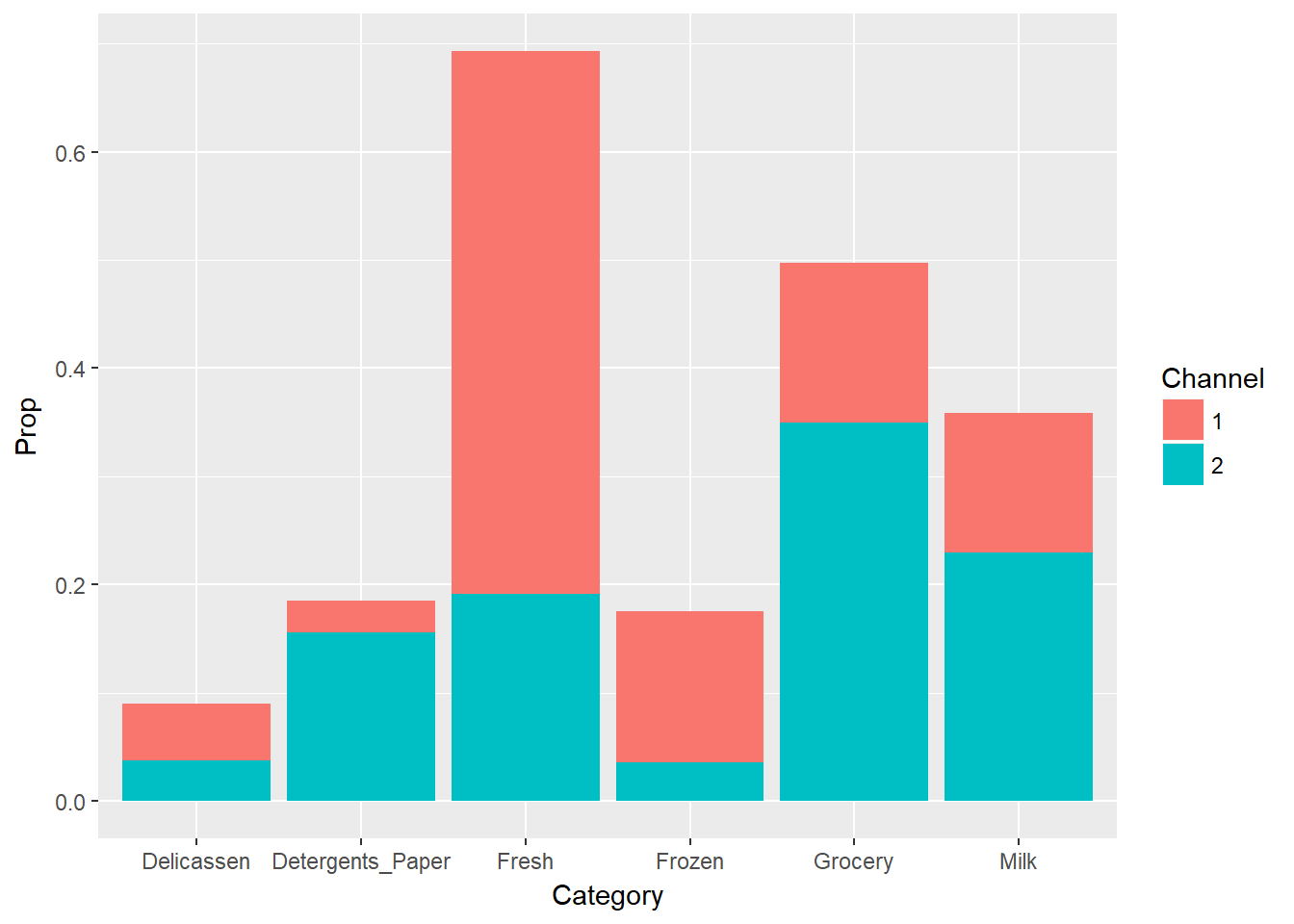Figure 4: Proportion of Spending per Channel

``````ggplot(data = customer_prop_region, aes(x = Category, y = Prop, fill = Region)) +
geom_bar(stat = "identity")``````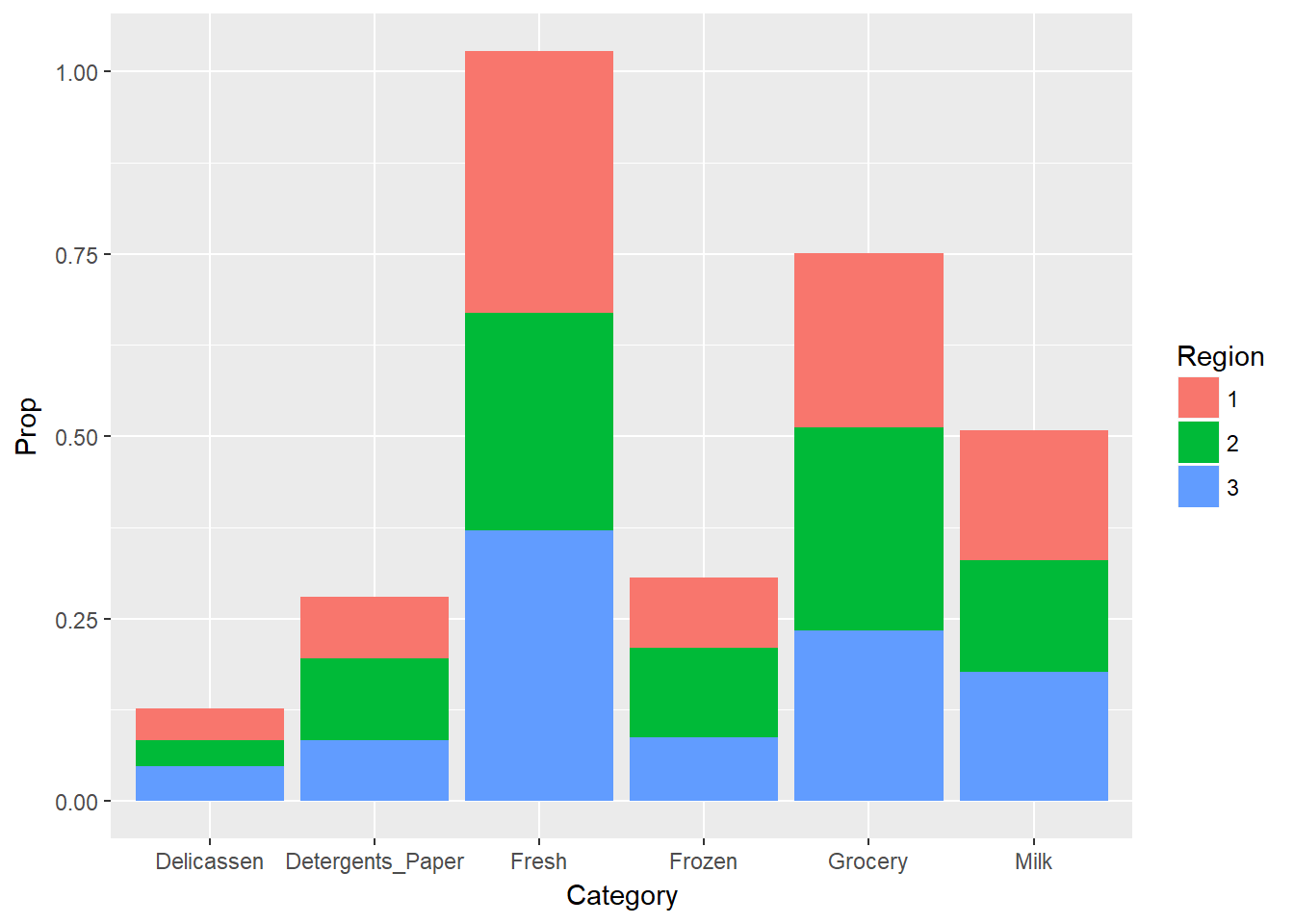Figure 5: Proportion of Spending per Region

From exploring the data, it seems more spending in channel 1 (horeca) goes to fresh and frozen products and more spending in channel 2 (retail) goes to grocery and detergents/paper. Also, The proportions of spending by region match those of by channel, indicating that there is likely not as much variation by region than there is by channel.

# Data Preprocessing

### Normalization

Because K-means and HCA both use measures of distance, the data need to be normalized so one feature does not dominate. However, the ‘Channel’ and ‘Region’ features should be handled separately from the others for two reasons:

• These features are categorical and the most dissimilar to the rest
• The other features all share the same unit of measurement, in this case, monetary units (m.u.)

Therefore, ‘Channel’ and ‘Region’ should either be normalized separately or turned into dummy variables. All of the other features will be normalized relative to each other.

``````# Returns a normalized vector between max / min
normalize <- function(x, max, min) {
return ((x - min) / (max - min))
}``````

``````# Normalize Channel and Region
customer_norm <- customer
customer_norm\$Channel <- normalize(customer\$Channel, max(customer\$Channel), min(customer\$Channel))
customer_norm\$Region <- normalize(customer\$Region, max(customer\$Region), min(customer\$Region))

# Get a subset of the data without 'Channel' and 'Region'
customer_products <- subset(customer_norm, select = c(-Channel, -Region))
str(customer_products)``````
``````## Classes 'data.table' and 'data.frame':   440 obs. of  6 variables:
##  \$ Fresh           : int  12669 7057 6353 13265 22615 9413 12126 7579 5963 6006 ...
##  \$ Milk            : int  9656 9810 8808 1196 5410 8259 3199 4956 3648 11093 ...
##  \$ Grocery         : int  7561 9568 7684 4221 7198 5126 6975 9426 6192 18881 ...
##  \$ Frozen          : int  214 1762 2405 6404 3915 666 480 1669 425 1159 ...
##  \$ Detergents_Paper: int  2674 3293 3516 507 1777 1795 3140 3321 1716 7425 ...
##  \$ Delicassen      : int  1338 1776 7844 1788 5185 1451 545 2566 750 2098 ...
##  - attr(*, ".internal.selfref")=<externalptr>``````

``````# Get the maximum and minimum of all features except 'Channel' and 'Region'
max_mu <- max(customer_products)
min_mu <- min(customer_products)

# Normalize the other features
customer_norm\$Fresh <- normalize(customer\$Fresh, max_mu, min_mu)
customer_norm\$Milk <- normalize(customer\$Milk, max_mu, min_mu)
customer_norm\$Grocery <- normalize(customer\$Grocery, max_mu, min_mu)
customer_norm\$Frozen <- normalize(customer\$Frozen, max_mu, min_mu)
customer_norm\$Detergents_Paper <- normalize(customer\$Detergents_Paper, max_mu, min_mu)
customer_norm\$Delicassen <- normalize(customer\$Delicassen, max_mu, min_mu)

# Check the data
lapply(customer_norm, summary)``````
``````## \$Channel
##    Min. 1st Qu.  Median    Mean 3rd Qu.    Max.
##  0.0000  0.0000  0.0000  0.3227  1.0000  1.0000
##
## \$Region
##    Min. 1st Qu.  Median    Mean 3rd Qu.    Max.
##  0.0000  0.5000  1.0000  0.7716  1.0000  1.0000
##
## \$Fresh
##    Min. 1st Qu.  Median    Mean 3rd Qu.    Max.
## 0.00000 0.02786 0.07580 0.10698 0.15097 1.00000
##
## \$Milk
##      Min.   1st Qu.    Median      Mean   3rd Qu.      Max.
## 0.0004637 0.0136427 0.0323144 0.0516573 0.0640872 0.6553394
##
## \$Grocery
##    Min. 1st Qu.  Median    Mean 3rd Qu.    Max.
## 0.00000 0.01917 0.04238 0.07087 0.09499 0.82727
##
## \$Frozen
##      Min.   1st Qu.    Median      Mean   3rd Qu.      Max.
## 0.0001962 0.0065917 0.0135803 0.0273650 0.0316657 0.5427293
##
## \$Detergents_Paper
##     Min.  1st Qu.   Median     Mean  3rd Qu.     Max.
## 0.000000 0.002263 0.007254 0.025667 0.034945 0.364019
##
## \$Delicassen
##     Min.  1st Qu.   Median     Mean  3rd Qu.     Max.
## 0.000000 0.003613 0.008582 0.013570 0.016204 0.427471``````

# K-Means

### Ensure the Results are Reproducible

``set.seed(77)``

### Find the Optimal Number of Clusters

K-means requires the number of clusters be specified. However, there is not enough domain logic or a business driven goal to help decide the number of clusters to try. Many different values for K can be tried and tested against each other using a measure of error. In this case, the ‘within cluster sum of squares.’

``````# Returns the tot.withinss of clusters with different k
get.k <- function(data, max_k) {
# Aggregate results
k <- c()
e <- c()

for (i in 1:max_k){
# Run K-means
cluster <- kmeans(data, iter.max = 1000, centers =  i)

# Get tot.withinss
error <- cluster\$tot.withinss

# Aggregate results
k <- c(k, i)
e <- c(e, error)
}

return (as.data.frame(list("K" = k, "Error" = e)))
}``````

The best K is one that generalizes well. The position of this is at the ‘elbow’ of where the error improvements trail off, suggesting diminishing returns.

``````# Get the K and error values
k_values <- get.k(customer_norm, 20)

# Plot
ggplot(aes(x = K, y = Error), data = k_values) +
geom_line() +
scale_x_continuous(limits = c(0, 20), breaks = seq(0, 20, 1))``````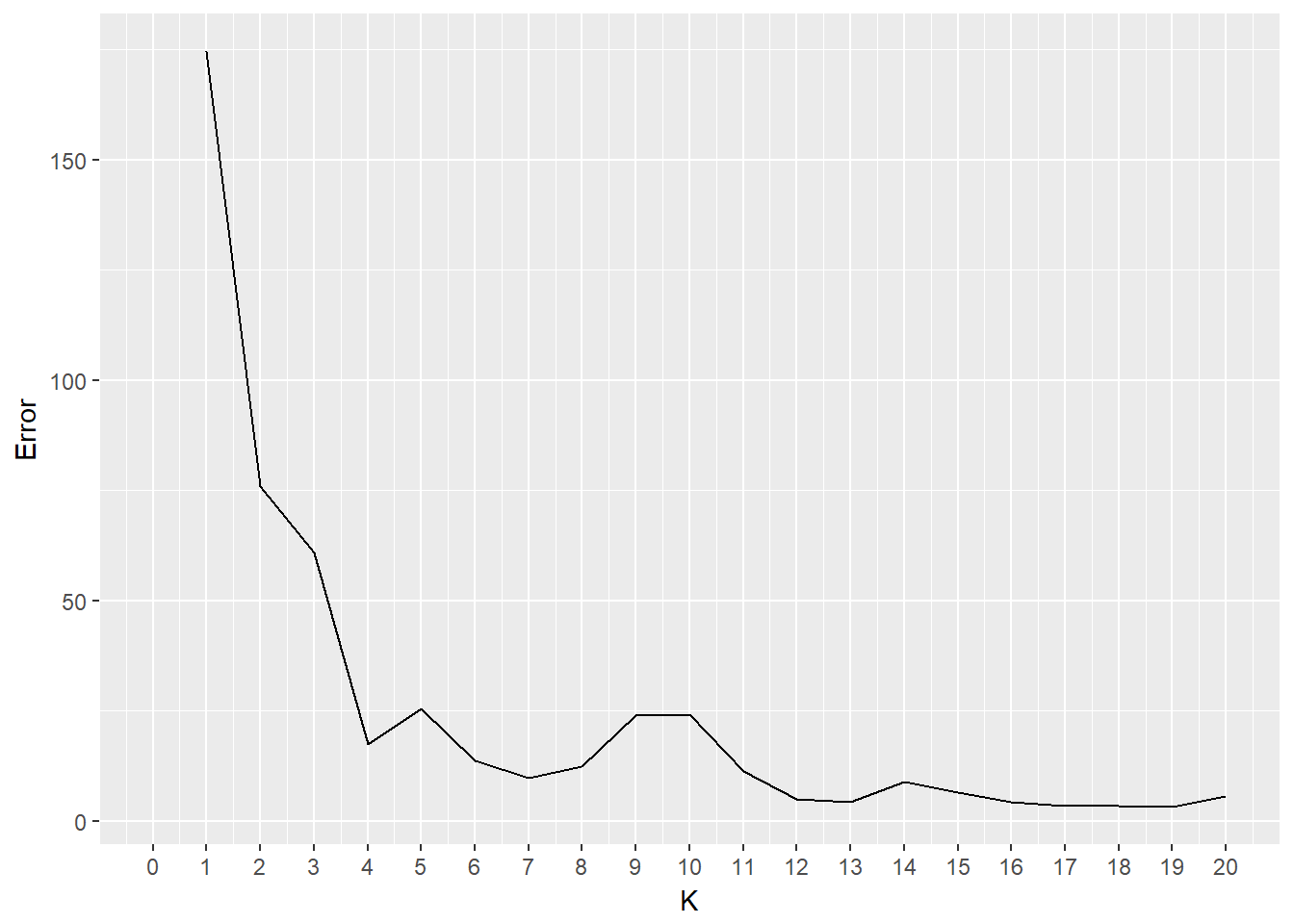Figure 6: K Value Error for All Data

The best K is either 3, 4, or 5. Leaving out the categorical features might give us different results.

``````# Get the K and error values
k_values <- get.k(subset(customer_norm, select = c(-Channel, -Region)), 20)

# Plot
ggplot(aes(x = K, y = Error), data = k_values) +
geom_line() +
scale_x_continuous(limits = c(0, 20), breaks = seq(0, 20, 1))``````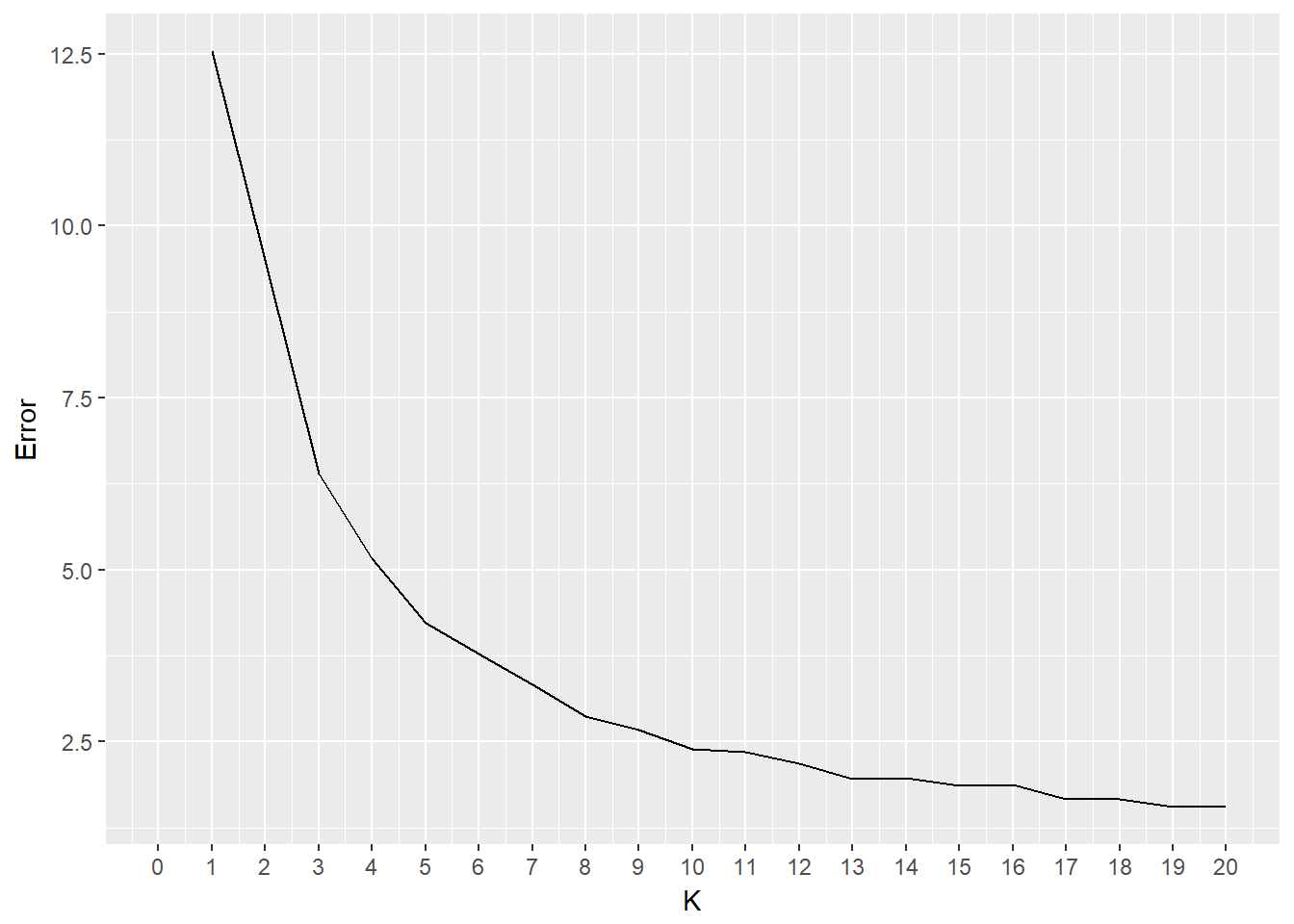Figure 7: K Value Error for Products Only

These results look much better, the error is lower. However, you could now argue that a K of 6 is now in the mix. Different values for K can be explored and conclusions can be drawn from the exploration

### Exploring The Clusters

Using a K of 3 and leaving out the categorical variables.

``customer_cluster <- kmeans(subset(customer_norm, select = c(-Channel, -Region)), iter.max = 1000, center = 3)``

##### Sizes

The size of each cluster can give an indication of how well K-means performed. However, even if sizes vary greatly, the data need to be explored further to determine if this is expected or useful. In this case, the smaller clusters probably relate to the outliers that were observed during the initial EDA step.

``````# Cluster sizes
customer_cluster\$size``````
``##   60  50 330``

##### Centers

The clusters centers can be analyzed as well. For each cluster, the average value of each product is calculated. In this case, large numbers indicate stronger relationships. For example, one could argue that cluster 1 represents Horeca more than it represents retail because it relates closer to the Fresh category and much less to the Detergents_Paper category. In retail, there is more emphasis on selling Grocery and Detergents_Paper, but perhaps less on Fresh and Frozen products. The third cluster is large, and seems to be less variant. This might be an indication that more clusters should be explored or that the outliers should be removed.

``````# Cluster sizes
customer_cluster\$centers``````
``````##        Fresh       Milk    Grocery     Frozen Detergents_Paper Delicassen
## 1 0.32045511 0.05387033 0.05604751 0.05984027      0.009243737 0.02716470
## 2 0.07130791 0.16503567 0.24584388 0.01777722      0.110607055 0.02005404
## 3 0.07356769 0.03407643 0.04705795 0.02291312      0.015783229 0.01011607``````

##### Clusters

By appending the cluster category to the original data frame some observations can be made more easily.

``````customer\$cluster <- as.factor(customer_cluster\$cluster)

# Fresh
aggregate(data = customer, Fresh ~ cluster, mean)``````
``````##   cluster    Fresh
## 1       1 35941.40
## 2       2  8000.04
## 3       3  8253.47``````

``````# Grocery
aggregate(data = customer, Grocery ~ cluster, mean)``````
``````##   cluster   Grocery
## 1       1  6288.617
## 2       2 27573.900
## 3       3  5280.455``````

##### Plotting

Getting a visualization of each cluster can provide some insight as well.

``ggplot(customer, aes(Grocery, Fresh, color = cluster)) + geom_point()``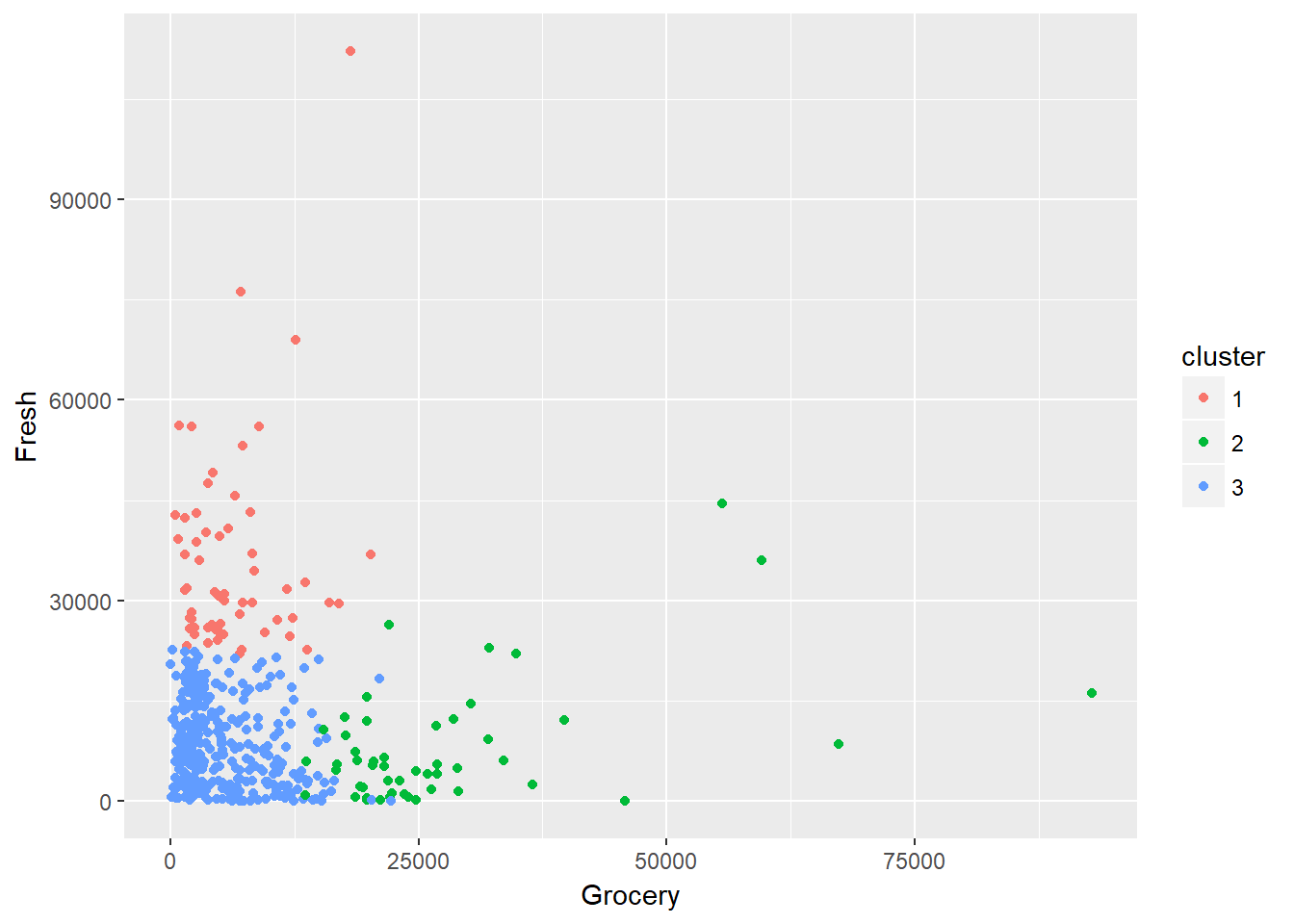Figure 8: Visualizing Clusters

# Hierarchical Cluster Analysis

### Find the Optimal Number of Clusters, Linkage, Distance Measurement

Determining the optimal number of clusters, linkage method, and distance measurement to use is tricky and requires domain knowledge. Visualizing different dendrograms could provide some insight, as can the `NbClust` package.

``````# Return the best K from a variety of methods
get.method <- function(data, methods) {
# Aggregate results
k <- c()
m <- c()

for (method in methods) {
# Run NbClust to find the best K
result <- NbClust(data = data, method = method)
best_k <- max(result\$Best.partition)

# Aggregate results
k <- c(k, best_k)
m <- c(m, method)
}

return (as.data.frame(list("K" = k, "Method" = m)))
}``````

``````# Running the following line produces messy output so it is commented out:
# results <- get.method(customer_norm, c("ward.D", "ward.D2", "single", "complete", "average", "mcquitty", "median", "centroid"))
# results
#    K   Method
# 1  7   ward.D
# 2  4  ward.D2
# 3  2   single
# 4  4 complete
# 5  2  average
# 6  4 mcquitty
# 7  2   median
# 8 11 centroid``````

### Visualizing ward.D2

``````customer_cluster <- hclust(dist(customer_norm), method="ward.D2")
plot(customer_cluster, hang = -0.001, cex = 0.001)``````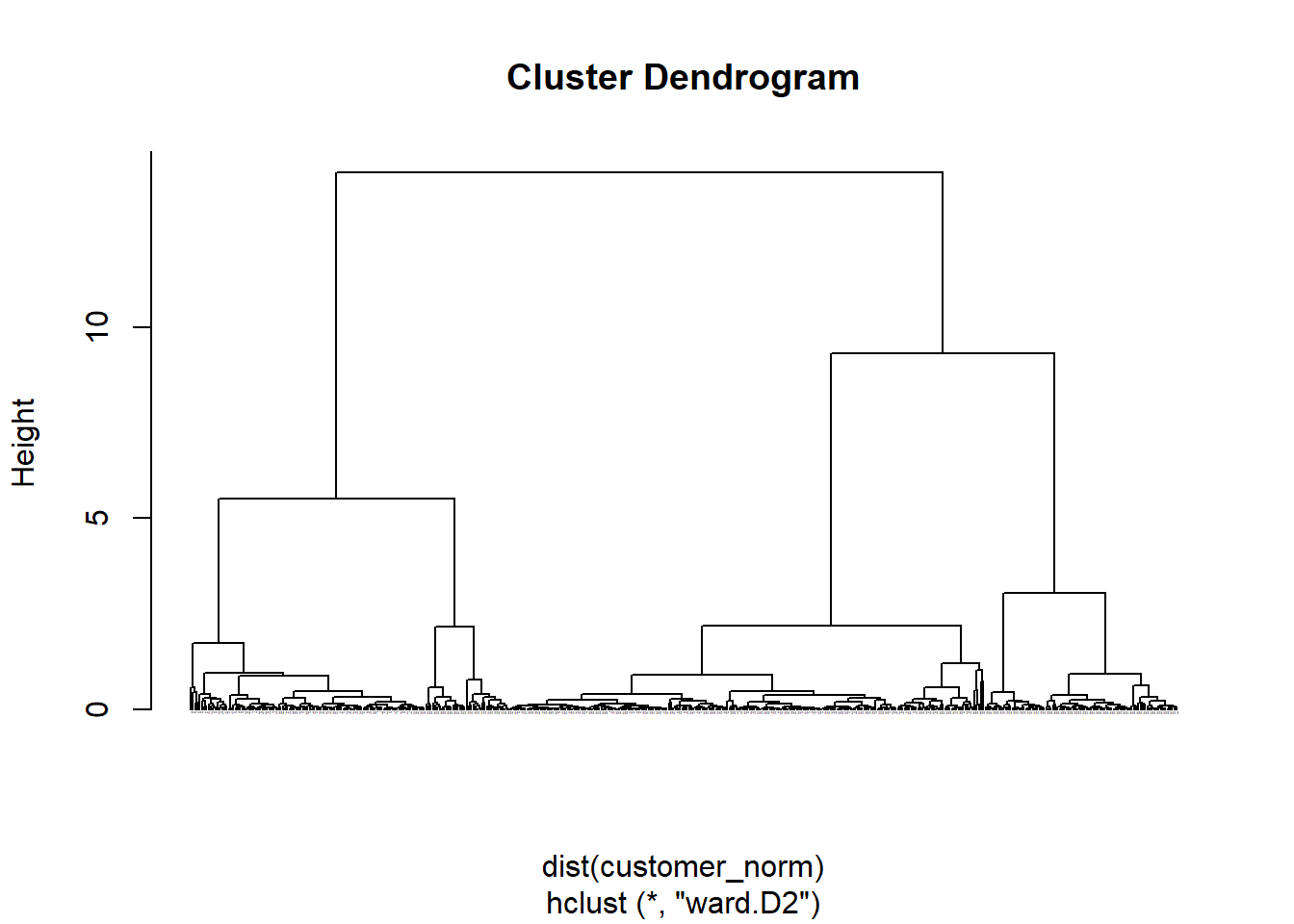Figure 9: ward.D2 Dendrogram

### Visualizing single

``````customer_cluster <- hclust(dist(customer_norm), method="single")
plot(customer_cluster, hang = -0.001, cex = 0.001)``````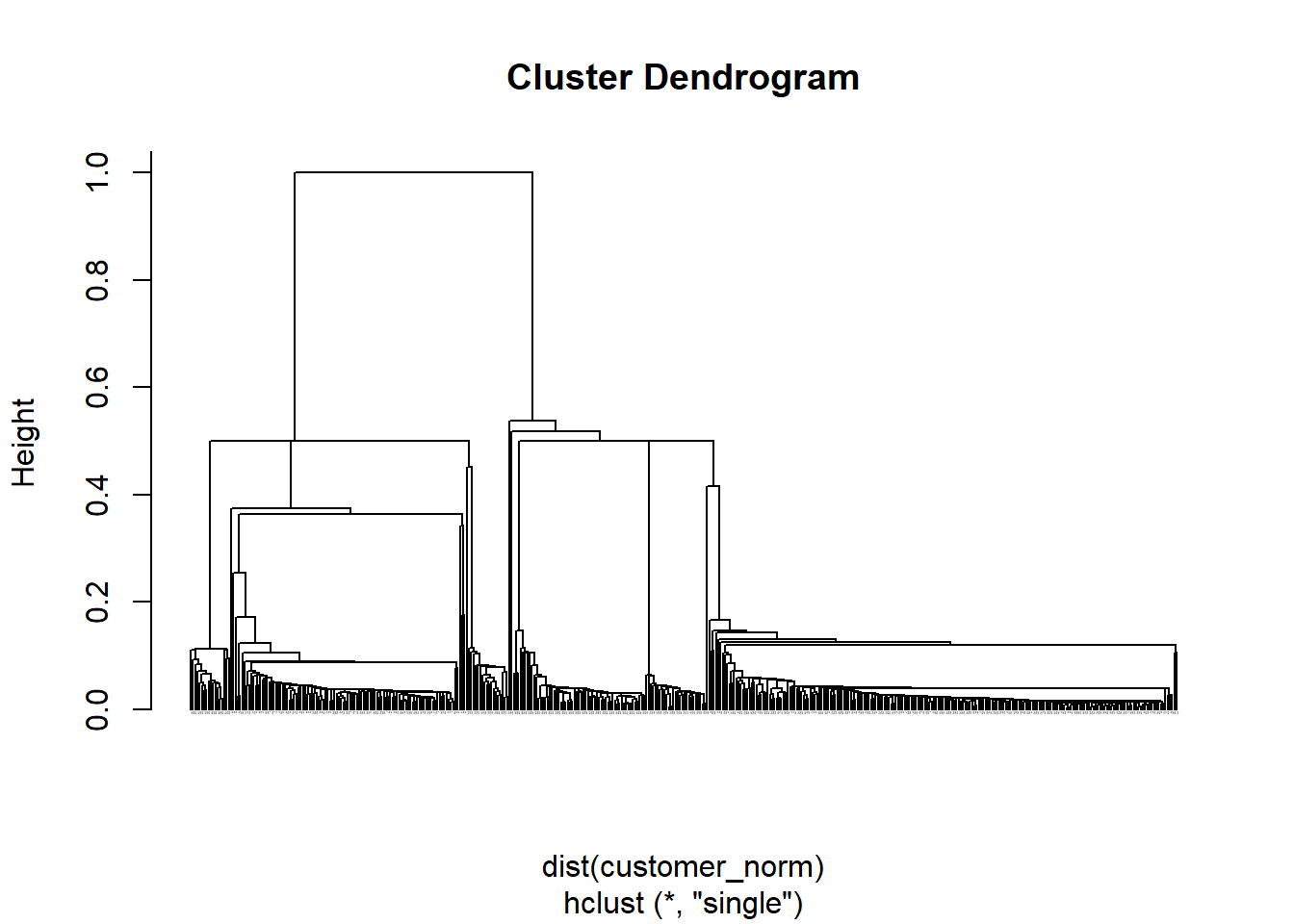Figure 10: single Dendrogram

### Visualizing complete

``````customer_cluster <- hclust(dist(customer_norm), method="complete")
plot(customer_cluster, hang = -0.001, cex = 0.001)``````